# The variation of displacement`
Question:

The variation of displacement with time of a particle executing free simple harmonic motion is shown in the figure.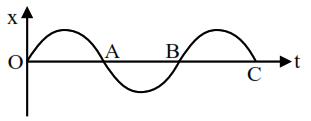The potential energy $U(x)$ versus time $(t)$ plot of the particle is correctly shown in figure :

1.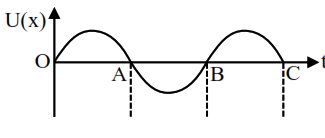2.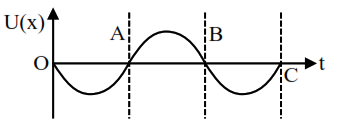3.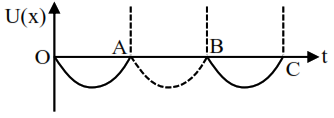4.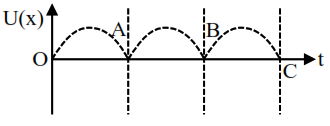Correct Option: , 4

Solution:

Potential energy is maximum at maximum distance from mean.